# Recent Trends in Nonlinear Evolution Equations

In recent years, there has been spectacular progress in the study of nonlinear diffusion equations and geometric flows. The fundamental aim of this workshop is to showcase these advances. Key challenges for the future that we target include the quantitative analysis of the dynamics of solutions arising from flows generated by deterministic, nondeterministic, and geometric evolution equations.

Tentative list of guest speakers of this workshop include:

• Ben Andrews (Australian National University)
• Yann Bernard (Monash University)
• Julie Clutterbuck (Monash University)
• James McCoy (University of Wollongong)
• EN Dancer (University of Sydney)
• Daniel Daners (University of Sydney)
• Jérôme Droniou (Monash University)
• Weiwei Ding (University of New England)
• Yihong Du (University of New England)
• David Galloway (University of Sydney)
• Zihua Guo (Monash University)
• Andree Lischewski (University of Adelaide)
• Pierre Portal (Australian National University)
• Derek Robinson (Australian National University)
• Melissa Tacy (University of Adelaide)
• Neil Trudinger (Australian National University)
• Xu-Jia Wang (Australian National University)
• Glen Wheeler (University of Wollongong)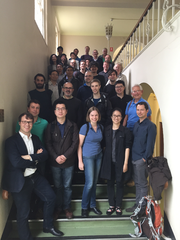During the two day workshop, talks from both communities are intertwined, serving to highlight salient ideas, proofs and questions, which are important fertile ground for pushing forward research in Australia and world-wide. In particular, this event has the intention to give PhD students and early career researchers the opportunity to meet some of the leading experts in the field of geometric evolution equations and nonlinear evolution equations. Every interested researcher is invited to attend and participate at this event. Please use Eventbrite to register for this workshop if you would like to attend (free). The Conference Dinner is on Wednesday evening (4 November 2015). If you want to attend the conference dinner, then please register for the dinner ($15 for Phd students and early career researchers,$30 for general participants). AMSI supports students and early career researchers without access to a suitable research grant or other sources, see below for further information.

## Venue

University of Sydney: Lecture Room 5, Physics Building. See also the information on how to get there.

## Program

Wednesday, 4 November 2015 Thursday, 5 November 2015
10:00 : Welcome
Chair: Daniel Hauer
10:05–10:40 : Fernando Quirós
Nonlocal filtration equations with rough kernels
10:45–11:05 : Coffee break
Chair: Yihong Du
11:05–11:40 : Xu-Jia Wang
Boundary estimates for singular elliptic equations
11:45–12:20 : Jérôme Droniou
Uniform-in-time stability result for doubly degenerate parabolic equations
12:25–14:00 : Lunch break at The Grandstand
Chair: Ben Andrews
14:05–14:40 : Derek Robinson
Uniqueness of diffusion on domains with rough boundaries
14:45–15:20 : Melissa Tacy
Bilinear $$L^{p}$$ estimates for quasimodes
15:25–15:40 : Coffee break
Chair: Jérôme Droniou
15:45–16:20 : Zihua Guo
Dichotomy of the solution to the 3D radial Zakharov system below the ground state
16:25–17:00 : James McCoy
The geometric triharmonic heat flow
17:05–17:40 : David Galloway
Instabilities of Dufort-Frankel finite differencing applied to coupled evolution equations

18:30 : Conference Dinner
09:00 : Welcome
Chair: Xu-Jia Wang
09:05–09:40 : EN Dancer
Some problems with large interaction
09:45–10:20 : Ben Andrews
Rolling stones in hyperspace
10:20–10:40 : Coffee break
Chair: EN Dancer
10:40–11:15 : Yihong Du
Constant solutions, ground-state solutions and radial terrace solutions
11:20–11:55 : Yann Bernard
Willmore-type problems: from subcritical to critical
12:00–13:40 : Lunch break at The Grandstand
Chair: Melissa Tacy
13:40–14:15 : Pierre Portal
Existence and uniqueness for non-autonomous parabolic systems with rough coefficients
14:20–14:55 : Daniel Daners
Perturbation of semi-linear evolution equations
15:00–15:20 : Coffee break
Chair: Yann Bernard
15:20–15:55 : Andree Lischewski
Cauchy problems for overdetermined geometric PDEs on Lorentzian manifolds and related Riemannian flow equations
16:00–16:35 : Weiwei Ding
Propagation phenomena of bistable reaction-diffusion equations in periodic habitats
16:40–17:15 : Glen Wheeler
Bottles for the inverse mean curvature flow

## Abstracts of Talks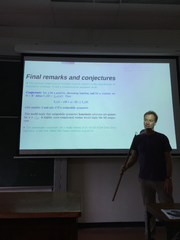### Rolling stones in hyperspace

Ben Andrews (Australian National University, Canberra)

#### Abstract

In 1974 William J Firey proposed a model for the changing shape of wearing stones in which the boundary surface moves with speed proportional to the Gauss curvature. He conjectured that the stones should become spherical as they shrink away to a point. I will discuss some of the history of this problem and a broader conjecture which generalises Fireys conjecture, and discuss some very recent progress (joint with Lei Ni and Pengfei Guan).

Back to Program### Willmore-type problems: from subcritical to critical

Yann Bernard (Monash University, Melbourne)

#### Abstract

Willmore immersions are critical points of the Willmore energy, which is the ${L}^{2}$ norm of the mean curvature of the immersion. They are weak solutions of a fourth-order nonlinear elliptic PDE and they are known to be smooth. A related problem investigates critical points of the the ${L}^{p}$ norm of the mean curvature, for $p>2$. This is an analytically easier problem in the sense that $p=2$ is the critical exponent and higher values of $p$ are subcritical. This talk will show how estimates that are uniform in $p$ can be developed and used to obtain information about Willmore immersions from information about solutions of the subcritical problem. This is an ongoing joint-work with Tristan Rivière, and, audience beware, it does $*$not$*$ involve an evolution equation.

Back to Program### Some problems with large interaction

EN Dancer (University of Sydney)

#### Abstract

We discuss results and open problems for the system

for large positive $k$, where $\Omega$ is an open subsets of ${ℝ}^{d}$. In particular, we discuss its relation with the scalar problem

and also the related parabolic problems.

Back to Program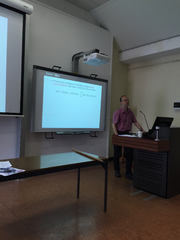### Perturbartion of semi-linear evolution equations

Daniel Daners (University of Sydney)

#### Abstract

Motivated by domain perturbation problems for parabolic initial boundary value problems, we discuss perturbation results for abstract semi-linear evolution equations in a Banach space. The main feature is that only very weak assumptions are needed at initial time. This requires a modification of the usual existence proof using a metric that does not force the inital condition to converge strongly. We then discuss lower semi-continuity of the existence time. The theory also implies the well known theory on parameter dependent equations.

Back to Program### Propagation phenomena of bistable reaction-diffusion equations in periodic habitats

Weiwei Ding (University of New England, Armidale)

#### Abstract

This talk is concerned with the propagation phenomena of spatially periodic reaction-diffusion equations with bistable nonlinearities. Firstly, we focus on the propagation solutions in the class of pulsating fronts. It is proved that, under various assumptions on the reaction terms, pulsating fronts exist when the spatial period is small or large. Furthermore, some properties of the set of the period for which there exist pulsating fronts with nonzero speed are established. We also characterize the sign of the front speeds and show the global exponential stability of the pulsating fronts with nonzero speed. Secondly, we investigate the propagation solutions in the larger class of transition fronts. It is shown that, under suitable assumptions, transition fronts reduce to pulsating fronts with nonzero wave speed. But the more interesting thing is that there exist new types of transition fronts which are not pulsating fronts. This is a joint work with François Hamel (AMU, France) and Xiao-qiang Zhao (MUN, Canada).

Back to Program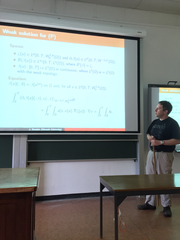### Uniform-in-time stability result for doubly degenerate parabolic equations

Jérôme Droniou (Monash University, Melbourne)

#### Abstract

Joint work with Robert Eymard (University Paris-Est Marne-la-Vallée) and Kyle Talbot (Monash University). We consider a class of doubly degenerate parabolic equations

 ${\partial }_{t}\left(\beta \left(u\right)\right)-\mathrm{div}\left(a\left(x,\nu \left(u\right),\nabla \zeta \left(u\right)\right)\right)=f$ (1)

with homogeneous Dirichlet boundary conditions. The functions $\beta$ and $\zeta$ are Lipschitz-continuous and non-decreasing, but may contain plateaux. The operator $-\mathrm{div}\left(a\left(x,s,\cdot \right)\right)$ is a Leray–Lions operator acting on ${W}_{0}^{1,p}\left(\Omega \right)$, and $\nu$ is defined by ${\nu }^{\prime }={\beta }^{\prime }{\zeta }^{\prime }$. Particular cases of (1) are:

Richards’ model of groundwater flow: ${\partial }_{t}\beta \left(u\right)-\mathrm{div}\left(K\left(x,\beta \left(u\right)\right)\nabla u\right)=f$. Here, $u$ is the pressure and $\nu \left(u\right)=\beta \left(u\right)$ is the saturation. Stefan’s model of melting material: ${\partial }_{t}u-\mathrm{div}\left(K\left(x,\zeta \left(u\right)\right)\nabla \zeta \left(u\right)\right)=f$. Here, $u$ is the internal energy and $\nu \left(u\right)=\zeta \left(u\right)$ is the temperature. Leray–Lions equations, with prototype the $p$-Laplace equation: ${\partial }_{t}u-\mathrm{div}\left(|\nabla u{|}^{p-2}\nabla u\right)=f$. Such models are involved in non-Newtonian filtration. Here, $\nu \left(u\right)=u$.

We present a stability result for the solutions of (1) under perturbations of the data $\beta ,\zeta ,a,f$, that is: if $\left({\beta }_{ϵ},{\zeta }_{ϵ},{a}_{ϵ},{f}_{ϵ}\right)$ converge as $ϵ\to 0$ to $\left(\beta ,\zeta ,a,f\right)$ in a natural sense, and if ${u}_{ϵ}$ is a solution to (1) with $\left({\beta }_{ϵ},{\zeta }_{ϵ},{a}_{ϵ},{f}_{ϵ}\right)$, then ${u}_{ϵ}\to u$ solution to (1). As usual, this stability result also gives the existence of solutions to the equation. The main novelty of our work is to establish the uniform-in-time and strong-in-space convergence (i.e. a $C\left(\left[0,T\right];{L}^{2}\left(\Omega \right)\right)$ convergence) of ${\nu }_{ϵ}\left({u}_{ϵ}\right)$. As demonstrated by the example above, $\nu \left(u\right)$ at a given time $t$ is the quantity of interest in the models. This uniform convergence is established under regularity assumptions on the data and the solutions that are compatible with applications – e.g. discontinuous permeabilities, etc.

The technique we use is based on an innovative combination of energy equalities (not mere estimates), compactness theorems (including compensated compactness), and convexity inequalities.

We will also show, through this study, that theoretical and numerical analyses of a PDE are not so far apart as one may imagine...

Back to Program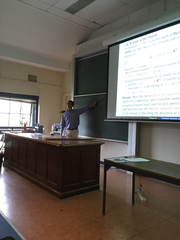### Constant solutions, ground-state solutions and radial terrace solutions

Yihong Du (University of New England, Armidale)

#### Abstract

In many applications, one is interested in the nonlinear parabolic problem

${u}_{t}-\Delta u=f\left(u\right)\left(x\in {ℝ}^{N},t>0\right),u\left(x,0\right)={u}_{0}\left(x\right)\left(x\in {ℝ}^{N}\right),$

where ${u}_{0}\in {L}^{\infty }\left({ℝ}^{N}\right)$ is nonnegative and has compact support, $f$ is a smooth function satisfying $f\left(0\right)=0$. One wants to know how much of the long-time dynamics of this problem is determined by the corresponding elliptic problem

$-\Delta u=f\left(u\right),u\ge 0\left(x\in {ℝ}^{N}\right).$

We show that, if $u\left(\cdot ,t\right)$ stays bounded in ${L}^{\infty }\left({ℝ}^{N}\right)$ for all $t>0$, then as $t\to \infty$, $u\left(\cdot ,t\right)$ converges to a stationary solution in ${L}_{loc}^{\infty }\left({ℝ}^{N}\right)$, provided that all the zeros of $f\left(u\right)$ in $\left[0,\infty \right)$ are nondegenerate (i.e., $f\left(u\right)=0$ and $u\ge 0$ imply ${f}^{\prime }\left(u\right)\ne 0$). Moreover, this stationary solution is either a stable constant solution (hence a stable zero of $f$), or a ground-state solution based on a stable zero of $f$ (namely a solution $v\left(x\right)$ of the elliptic problem which is radially symmetric about some point ${x}_{0}\in {ℝ}^{N}$, and decreases in $|x-{x}_{0}|$, with $\underset{|x|\to \infty }{lim}v\left(x\right)$ a stable zero of $f$). Thus, viewed in the space ${L}_{loc}^{\infty }\left({ℝ}^{N}\right)$, the long-time behavior of $u\left(\cdot ,t\right)$ is determined by two simplest types of solutions of the corresponding elliptic problem.

Furthermore, we show that, viewed in the space ${L}^{\infty }\left({ℝ}^{N}\right)$, the long-time behavior of $u\left(\cdot ,t\right)$ resembles that of a radial terrace solution $v\left(|x|,t\right)$, whose limit as $t\to \infty$ is determined completely by a propagating terrace of 1-space dimension, which by definition is a set of traveling wave solutions ${\left\{{w}_{i}\right\}}_{i=1}^{k}$, with each ${w}_{i}$ solving the elliptic equation

$-{w}_{zz}+{c}_{i}{w}_{z}=f\left(w\right)\left(z\in {ℝ}^{1}\right),$

where ${c}_{i}$ is a certain constant, known as the wave speed of ${w}_{i}$.

This talk is based on joint works with Peter Polacik and with Hiroshi Matano.

Back to Program### Instabilities of Dufort-Frankel finite differencing applied to coupled evolution equations

Dave Galloway (University of Sydney)

#### Abstract

Du Fort-Frankel is a tactic to stabilise Richardson’s unstable 3-level leapfrog time-stepping scheme. By including the next time level in the right hand side evaluation, it is implicit, but it can be re-arranged to give an explicit updating formula, thus apparently giving the best of both worlds. Textbooks prove unconditional stability for the heat equation, and extensive use on a variety of advection-diffusion equations has produced many useful results. Nonetheless, for some problems the scheme can fail in an interesting and surprising way, leading to instability at very long times. An analysis for a simple teaching problem involving a pair of evolution equations that describe the spread of a rabies epidemic gives insight into how this occurs. An even simpler modified diffusion equation suffers from the same instability. Finally the rabies problem is revisited and a way is found to fix things, although no prescriptive recipe is known which selects this particular choice.

Back to Program### Cauchy problems for overdetermined geometric PDEs on Lorentzian manifolds and related Riemannian flow equations

#### Abstract

In this talk we consider Lorentzian manifolds admitting a covariantly constant null vector field. Such vector fields are solutions of an overdetermined PDE arising naturally in general relativity and other geometric contexts. We show that the Cauchy problem for these geometries is well-posed, i.e. the manifolds under consideration can be constructed starting from an initial hypersurface using constraints and evolution equations. These evolution equations lead to the analysis of an interesting quasilinear symmetric hyperbolic PDE which couples the Ricci curvature with other geometric data and it generalizes the PDE system appearing in the Cauchy problem for the vacuum Einstein equations. The constraints imposed on the initial hypersurface can be reformulated in terms of flow equations for certain Riemannian metrics. As an application of this construction to Riemannian geometry, we give a classification of geometries admitting solutions to certain overdetermined first order PDEs for spinor fields.

Back to Program### The geometric triharmonic heat flow

James McCoy (University of Wollongong)

#### Abstract

We consider closed immersed surfaces in ${ℝ}^{3}$ evolving by the geometric triharmonic heat flow. Using local energy estimates, we prove interior estimates and a positive absolute lower bound on the lifespan of solutions depending solely on the local concentration of curvature of the initial immersion in ${L}^{2}$. We further use an $\epsilon$-regularity type result to prove a gap lemma for stationary solutions.

Using a monotonicity argument, we then prove that a blowup of the flow approaching a singular time is asymptotic to a non-umbilic embedded stationary surface.

This allows us to conclude that any solution with initial ${L}^{2}$-norm of the tracefree curvature tensor smaller than an absolute positive constant converges exponentially fast to a round sphere with radius equal to $\sqrt{3{V}_{0}∕4\pi }$, where ${V}_{0}$ denotes the signed enclosed volume of the initial data.

This is joint work with Scott Parkins and Glen Wheeler

Back to Program### Existence and uniqueness for non-autonomous parabolic systems with rough coefficients

Pierre Portal (Australian National University, Canberra)

#### Abstract

In the late 1950s, two schools obtained a series of results on non-autonomous linear parabolic equations with bounded measurable (in space and time) coefficients. Lions school, on the one hand, used form methods. They could handle systems, but had to work with ${L}^{2}$ data. Nash and Aronson, on the other hand, used heat kernel methods, and obtained regularity results that allowed them to handle ${L}^{p}$ data. Their method, however, could not be applied to systems. Ever since, results for systems and ${L}^{p}$ data have been limited to coefficients that are Holder regular in time. Pascal Auscher, Sylvie Monniaux, and I have developed, over the past four years, a new approach to the problem that lifts these limitations. For $p\ge 2$, we obtain existence and uniqueness results for systems with bounded measurable coefficients in space and time, and ${L}^{p}$ data. For a range of values of $p$ below $2$, we obtain existence and uniqueness results for ${L}^{p}$ data, and systems with coefficients that are $BV$ in time and bounded measurable in space.

Our approach has its origin in elliptic boundary value problems on rough domains. We consider our evolution equation as a boundary value problem in a rough space-time domain, and extend singular integrals, functional calculus, and Hardy space methods originally designed for elliptic BVP.

Back to Program### Uniqueness of diffusion on domains with rough boundaries

Derek W. Robinson (Australian National University, Canberra)

#### Abstract

We describe recent work with Juha Lehrbäck, University of Jyväskylä, on diffusion processes related to an elliptic form $h$ on a domain $\Omega$ in ${ℝ}^{d}$ with ‘rough’ boundary. We assume $h\left(\phi \right)={\sum }_{k,l=1}^{d}\left({\partial }_{k}\phi ,{c}_{kl}{\partial }_{l}\phi \right)$ with domain ${C}_{c}^{\infty }\left(\Omega \right)$ where the ${c}_{kl}$ are real symmetric ${L}_{\infty }\left(\Omega \right)$-functions with $C\left(x\right)=\left({c}_{kl}\left(x\right)\right)>0$ for almost all $x\in \Omega$. We further assume that there are $a,\delta >0$ such that ${a}^{-1}{d}_{\Gamma }^{\phantom{\rule{3.26288pt}{0ex}}\delta }\phantom{\rule{0.3em}{0ex}}I\le C\le a\phantom{\rule{0.3em}{0ex}}{d}_{\Gamma }^{\phantom{\rule{3.26288pt}{0ex}}\delta }\phantom{\rule{0.3em}{0ex}}I$ for ${d}_{\Gamma }\le 1$ where ${d}_{\Gamma }$ is the Euclidean distance to the boundary $\Gamma$ of $\Omega$. The degeneracy of the coefficients impedes the diffusion near the boundary and if the degeneracy is sufficiently strong then the boundary is out of reach. Therefore one would expect a unique diffusion process. We establish that this is the case if and only if $\delta \ge 1+\left(s-\left(d-1\right)\right)$ where $s$ is the Hausdorff dimension of $\Gamma$. The main assumption is Ahlfors $s$-regularity of the boundary and if $s\ge d-1$ we also assume a mild uniformity property for $\Omega$ in the neighbourhood of one $z\in \Gamma$.

Our results apply to forms on Lipschitz domains or on a wide class of domains with $\Gamma$ a self-similar fractal. In particular it applies to the interior or exterior of the von Koch snowflake curve in ${ℝ}^{2}$ or the complement of a uniformly disconnected set in ${ℝ}^{d}$.

Back to Program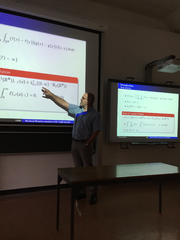### Nonlocal filtration equations with rough kernels

#### Abstract

We study the problem with nonlocal diffusion

${\partial }_{t}u+L\phi \left(u\right)=0,$

posed for $x\in {ℝ}^{N}$, $t>0$, where $L$ is a Lévy-type non-local operator with a singularity at the origin as that of the fractional Laplacian, and $\phi$ is a nondecreasing continuous function, with an initial data in ${L}^{1}\left({ℝ}^{N}\right)$. We prove existence and uniqueness of weak solutions. For a wide class of nonlinearities $\phi$, including the porous media case, these solutions turn out to be bounded and ${C}^{\alpha }$ continuous for $t\ge \tau$ for any $\tau >0$. We also describe the asymptotic behaviour when the nonlinearity resembles a power for $u\approx 0$ and $L$ is close to the fractional Laplacian. Joint work with Arturo de Pablo and Ana Rodríguez.

Back to Program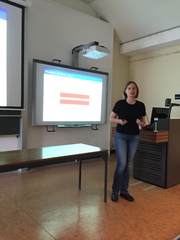### Bilinear ${L}^{p}$ estimates for quasimodes

#### Abstract

Understanding the growth of the product of eigenfunctions

$u\cdot v$

$\Delta u=-{\lambda }^{2}u\phantom{\rule{1em}{0ex}}\Delta v=-{\mu }^{2}v$

is vital to understanding the regularity properties of non-linear PDE such as the non-linear Schrödinger equation. In this talk I will discuss some recent results that I have obtain in collaboration with Zihua Guo and Xiaolong Han which provide a full range of estimates of the form

$\parallel uv{\parallel }_{{L}^{p}}\le G\left(\lambda ,\mu \right)\parallel u{\parallel }_{{L}^{2}}\parallel v{\parallel }_{{L}^{2}}$

where $u$ and $v$ are approximate eigenfunctions of the Laplacian. We obtain these results by re-casting the problem to a more general related semiclassical problem.

Back to Program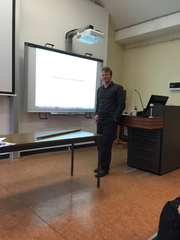### Bottles for the inverse mean curvature flow

Glen Wheeler (University of Wollongong)

#### Abstract

In this talk I describe recent joint work with Hojoo Lee (KIAS, South Korea) and Gregory Drugan (U Washington, United States) in which we investigate special solitons for the inverse mean curvature flow that we term ”bottles”.

Back to Program### Boundary estimates for singular elliptic equations

Xujia Wang (Australian National University, Canberra)

#### Abstract

Elliptic equations with singularities near the boundary arise in a number of applications. In this talk we discuss the optimal regularity and higher order singularity profile near the boundary.

Back to Program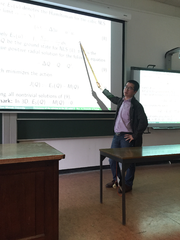### Dichotomy of the solution to the 3D radial Zakharov system below the ground state

Zihua Guo (Monash University, Melbourne)

#### Abstract

We consider the 3D Zakharov system in the radial case. We obtain dichotomy (blowup and scattering) of the solutions below the ground state. The method relies on the concentration-compactness/rigidity method developed by Kenig-Merle, and normal form method and radial-improved Strichartz estimates.

Back to Program

## Travel Support available from AMSI

This event is sponsored by the Australian Mathematical Sciences Institute (AMSI). AMSI allocates a travel allowance annually to each of its member universities. Students or early career researchers from AMSI member universities without access to a suitable research grant or other source of funding may apply to the Head of Mathematical Sciences for subsidy of travel and accommodation out of the departmental travel allowance. For applications to travel fundings please see research.amsi.org.au/travel-funding.